StatLect

# Realization of a random variable

The value that a random variablewill take is, a priori, unknown. However, after we receive the information thathas taken a certain value(i.e.,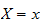), the valueis called the realization of.

The concept extends in the obvious manner also to random vectors and random matrices.## Notation

Note that random variables are usually denoted by an uppercase letter, while their realizations are usually denoted by a lowercase letter.

So, for example, if we denote a random variable by, its realization is denoted by.

Remember that random variables are functions defined on the set of all possible outcomes of a probabilistic experiment, which is denoted byand is called sample space.

If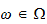is the realized outcome, that is, the outcome that has actually happened, then the value taken by the random variable, which is associated to that outcome, is denoted by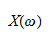Also in this case we say that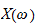is the realization of.

## Example

Suppose that we perform a probabilistic experiment having two possible outcomes,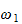and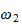.

The sample space, that is, the set of all possible outcomes, is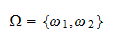Now, suppose that we have a random variabledefined as follows: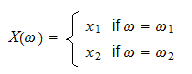If the realized outcome of the experiment is, then the realization of the random variableis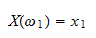## More details

For more details about the realization of a random variable, you can read the lecture entitled Random variables.

Previous entry: Random matrix

Next entry: Robust standard errors